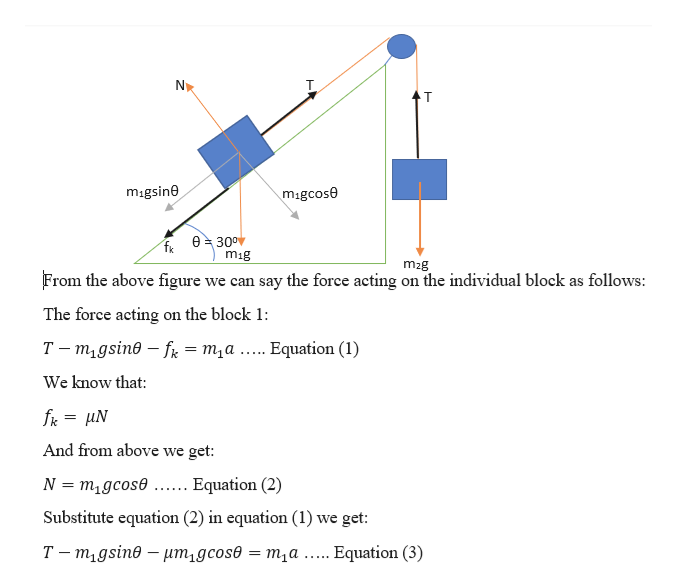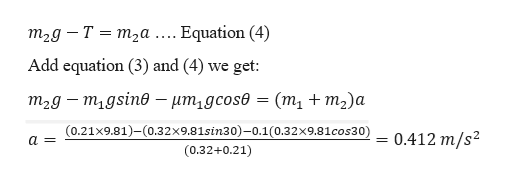# and Tests- Eric LeonXGd. Present and work an22434090s interval. How do the magnitudes of F, and F2 compare? (Neglect theTip 6S.P0soln.wersSsidA block of mass m,-320 g is at rest on a plane that makes an angle e 30° above the horizontal. The coefficient of kinetic friction between the block and the plane issecond block of mass m,-210 g that hangs freely by a string that passes over a frictionless and massless pulley. Find its speed when the second block has fallen 30.0 cm34X cm/sa coordinate system in which the +x direction is up the incline for the block on the incline and downward for the harnging block. Draw free body diagrams of the ford the two forces acting on the hanging block. Because they are attached tby the string, the blocks will have a common acceleration. Apply Newton's second adetermine the acceleration of the system, Kinematic expressions can then be used to determine the speed.Practice Another VersionTipler6 5.P.052evious Answers

Question
33 views

Uk=0.10 but it's not in the picturehelp_outlineImage Transcriptioncloseand Tests- Eric Leon X G d. Present and work an 22434090 s interval. How do the magnitudes of F, and F2 compare? (Neglect the Tip 6S.P0soln. wers Ssid A block of mass m,-320 g is at rest on a plane that makes an angle e 30° above the horizontal. The coefficient of kinetic friction between the block and the plane is second block of mass m,-210 g that hangs freely by a string that passes over a frictionless and massless pulley. Find its speed when the second block has fallen 30.0 cm 34 X cm/s a coordinate system in which the +x direction is up the incline for the block on the incline and downward for the harnging block. Draw free body diagrams of the for d the two forces acting on the hanging block. Because they are attached tby the string, the blocks will have a common acceleration. Apply Newton's second a determine the acceleration of the system, Kinematic expressions can then be used to determine the speed. Practice Another Version Tipler6 5.P.052 evious Answers fullscreen
check_circle

Step 1

Given information:

Mass of the block 1 (m1) = 320 g = 0.32 kg

Mass of the block 2 (m2) = 210 g =0.21 kg

Angle of inclination (θ) = 300

Coefficient of kinetic friction (µk) = 0.10

Height the block 2 has fallen (h) = 30 cm = 0.3 m

Step 2help_outlineImage TranscriptioncloseNX migsine migcose e 30° mig m2g From the above figure we can say the force acting on the individual block as follows: The force acting on the block 1: T m1gsin f = m,a ..... Equation (1) We know that fk иN And from above we get: N migcose Equation (2) Substitute equation (2) in equation (1) we get Т-т,gsing — иm,gcose —D т,а ..... Equation (3) fullscreen
Step 3

The force acting on th...help_outlineImage Transcriptioncloseт2д — Т %3D т,а . Equation (4) Add equation (3) and (4) we get: т,д — т,gsine - ит,дсos® — (т,+m)a (0.21x9.81)-(0.32x9.81sin3 0) -0.1 (0.32x9.8 1 cos3 0) 0.412 m/s2 a= (0.32+0.21) fullscreen

### Want to see the full answer?

See Solution

#### Want to see this answer and more?

Solutions are written by subject experts who are available 24/7. Questions are typically answered within 1 hour.*

See Solution
*Response times may vary by subject and question.
Tagged in
SciencePhysics

### Newtons Laws of Motion